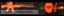-=+=- -=+=- -=+=- -=+=- -=+=- -=+=- -=+=- -=+=- -=+=- -=+=- -=+=- -=+=- -=+=- -=+=- -=+=- -=+=- -=+=- -=+=- -=+=- -=+=- -=+=- -=+=- -=+=- -=+=- -=+=- -=+=- -=+=- -=+=- -=+=- -=+=- (c) WidthPadding Industries 1987 0|433|0 -=+=- -=+=- -=+=- -=+=- -=+=- -=+=- -=+=- -=+=- -=+=- -=+=- -=+=- -=+=- -=+=- -=+=- -=+=- -=+=- -=+=- -=+=- -=+=- -=+=- -=+=- -=+=- -=+=- -=+=- -=+=- -=+=- -=+=- -=+=- -=+=- -=+=-
SoCoder -> Blogs Home -> Blogs

svrmanCreated : 21 February 2007
Edited : 10 September 2007

Page scraping article done!

|edit| It's now back up!

alternatively

Enjoy!
|edit|

|edit| This blog entry was posted some time ago and unfortunately the bb.com rss script and article are no longer online.
I may host these up in the future on my site at a later date.
- noted july 2007
|edit|

I had a long winded post I wanted to put up here but had some initial problems posting (was getting 401 errors) so I saved it to a text file so I could post later.

When I looked at it again, I made a few amendments and just added to the original post to make it into a sort of article.
so here's the finished piece:

Well I just wanted to share it with you guysFeedback welcome.

Screenshots:peace.

Wednesday, 21 February 2007, 17:32
power mouseyI uh read a little of it.
Lets just say when I'm more cognant, conscious and more awake I'll read it further.

Sorry.but cheersWednesday, 21 February 2007, 17:42
JayenkaiThat's not gonna be good for bandwidth!

On a related note, RSS feeds on the way, they're messing up in Google Reader though, so I want to keep tweaking them till they're right.Wednesday, 21 February 2007, 18:12
svrmanYeh lol but I doubt we could bring them down just by using that script!
Nah, I haven't really analysed the bandwidth cost from using this script but I don't think it'd be anymore if I was go to the site and refresh every 2-3 minutes, which I do sometimes! XD

Good to hear you'll be using rss feeds soon.Wednesday, 21 February 2007, 18:57
Jayenkai